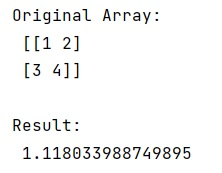# How does numpy.std() method work?

Learn about the numpy.std() method in Python, how does it work?
Submitted by Pranit Sharma, on January 10, 2023

NumPy is an abbreviated form of Numerical Python. It is used for different types of scientific operations in python. Numpy is a vast library in python which is used for almost every kind of scientific or mathematical operation. It is itself an array which is a collection of various methods and functions for processing the arrays.

## Python numpy.std() method

The numpy.std() method computes the standard deviation along the specified axis and it returns the standard deviation, a measure of the spread of a distribution, of the array elements. The standard deviation is computed for the flattened array by default, otherwise over the specified axis.

It takes an array to calculate the standard deviation of these values. Also, it takes an argument called Axis or axes. It is the axis along which the standard deviation is computed. The default is to compute the standard deviation of the flattened array.

The standard deviation is the square root of the average of the squared deviations from the mean, i.e., std = sqrt(mean(x)), where x = abs(a - a.mean())**2.

The average squared deviation is typically calculated as x.sum() / N, where N = len(x). If, however, ddof is specified, the divisor N - ddof is used instead.

Let us understand with the help of an example,

## Python code to demonstrate the example of numpy.std() method

```# Import numpy
import numpy as np

# Creating an array
arr = np.array([[1, 2], [3, 4]])

# Display original array
print("Original Array:\n",arr,"\n")

# Using std method
res = np.std(arr)

# Display result
print("Result:\n",res,"\n")
```

Output: